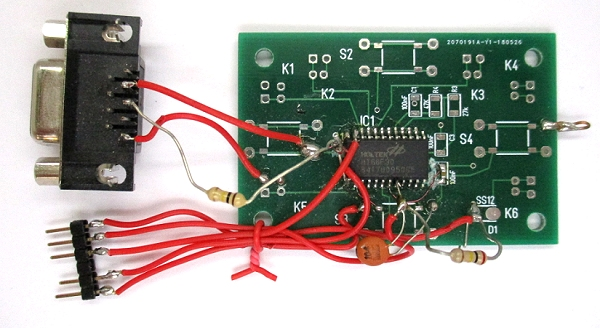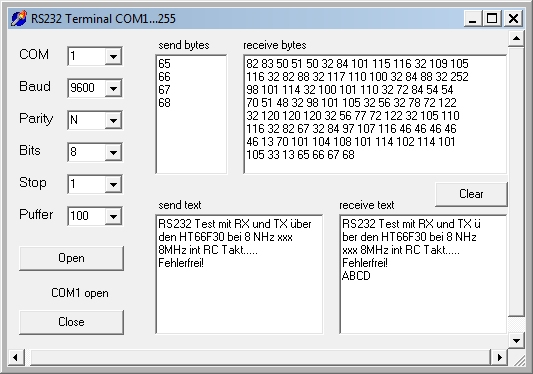# Serielle Schnittstelle für den HT66F30Der Holtek HT66F30 hat von sich aus keine serielle Schnittstelle. Aber für das Debuggen kann man auf jeden Fall eine gebrauchen. Das Beispiel von Hermann Nieder für den HT46F47 war in Assembler geschrieben. Unter den C-Beispielen des Compilers fand sich aber auch das Projekt Serial. Es ließ sich leicht für den HT66F30 anpassen. Außerdem habe ich für die Funktionen tansmit und receive jeweils eine invertierte Version  erzeugt, die ohne zusätzliche Hardware direkt an einer RS232 angeschlossen werden kann. In dem Fall ist nur ein Widerstand von 100 k in der RX-Leitung nötig.

`void transmit(unsigned char);void transmit_inv(unsigned char);void receive(unsigned char *);void receive_inv(unsigned char *);`

`#include "HT66F30.h"//Serial.c////Mask option//WDT : Disable//the others use the default value#pragma vector isr_4 @ 0x4#pragma vector isr_8 @ 0x8#pragma vector isr_c @ 0xc//ISR for safequardvoid isr_4(){} // external ISRvoid isr_8(){} // timer/event 0void isr_c(){} // timer/event 1#define tx   _pa1               //transmit pin#define rx   _pa0               //receive pinunsigned char data;unsigned char vall @0x20;void transmit(unsigned char);void transmit_inv(unsigned char);void receive(unsigned char *);void receive_inv(unsigned char *);//system frequency: 4MHz//#define T 104 //baudrate 19200 = 8M/4/(T) => T = 104#define T 208 //baudrate 9600 = 8M/4/(T) => T = 208//#define T 416 //baudrate 4800 = 8M/4/(T) => T = 416//#define T 832 //baudrate 2400 = 8M/4/(T) => T = 832 void transmit(unsigned char val){        unsigned char i;                tx = 0;       _delay(T-14);        for(i=0; i<8; i++)  {            if (val & 1)     tx = 1;            else     tx = 0;            val >>= 1;         _delay(T-14);        }        tx = 1;        _delay(T);}void transmit_inv(unsigned char val){        unsigned char i;                tx = 1;       _delay(T-14);        for(i=0; i<8; i++)  {            if (val & 1)     tx = 0;            else     tx = 1;            val >>= 1;         _delay(T-14);        }        tx = 0;        _delay(T);} void receive(unsigned char *val){   unsigned char i,v;   v = 0;   while(rx);    //wait start bit   _delay(T);      _delay(T/2);  //     for(i=0; i<8; i++)  {        v >>= 1;        if (rx)           v |= (unsigned char)0x80;         _delay(T-14); //     }    *val=v;}void receive_inv(unsigned char *val){   unsigned char i,v;   v = 0;   while(!rx);    //wait start bit   _delay(T);      _delay(T/2);  //     for(i=0; i<8; i++)  {        v >>= 1;        if (!rx)           v |= (unsigned char)0x80;         _delay(T-14); //     }    *val=v;}//a long time delayvoid mydelay(unsigned int times){	while(times--)	_delay(65000);}void main(){	unsigned char i, j, idx;	_pbc = 0;	_pb = 85;	_acerl = 0;  //Port a kein AD-Input sondern Port	_cp0c =0;  // Conparator frei geben	_cp1c =0;  // Conparator frei geben	_pac = 0; //set port A to output port	_pa = 0;  //zero port A 		_pac0 = 1;      //set receive pin to input mode    _pac1 = 0;      //set transmit pin to output mode 	tx = 0;        while(1)  {                receive_inv(&data);				//data = 85;                transmit_inv(data);                // _delay(50000);        }		//	_papu = 255; //pullup	while(1) {			_pb = 85;			_pa = 85;			_delay(1000);			_pb = 170;			_pa = 170;			_delay(1000);		}}`

Ein Test mit Terminal.exe zeigt eine korrekte Übertragung der empfangenen Zeichen. Obwohl  für den internen RC-Oszillator 5 V vorgesehen ist, läuft die Übertragung auch bei 3 V noch fehlerfrei.